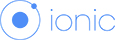## Javascript get compass direction with the two longitude and latitude

### Tagged Under : IONIC, JavascriptJust develop a project with IONIC and google map to tracking the transport routing. Because the google map wouldn’t rotate itself when the transport moving. It will confuse the user when they look at the map.

So I need to rotate the map to let navigation always facing to top. It will easy user to understand when they look at the map. To calculate the compass direction we need 2 longitude and latitude. that is previous and current longitude latitude.
Below is the script to calculate the compass direction:
```
getDirection(lat1, lng1, lat2, lng2) {
var PI = Math.PI;
var dTeta = Math.log(Math.tan((lat2/2)+(PI/4))/Math.tan((lat1/2)+(PI/4)));
var dLon = Math.abs(lng1-lng2);
var teta = Math.atan2(dLon,dTeta);
var direction = Math.round((teta * 180 / Math.PI));
return direction;
}
```